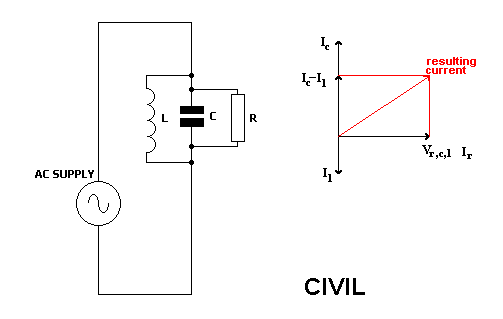Home > Electronic Tutorials > Phasors and Resonance Tutorial > L, C and R in Parallel in AC Circuits Tutorial

Electronic Tutorials - Phasors and Resonance

# L, C and R in Parallel in AC Circuits TutorialSince all components are in parallel, the same voltage is across all of them.

This is indicated by the horizontal phasor.

Resistor current and voltage are in phase, so resistor current is also along this phasor.

CIVIL tells us that capacitor current leads the voltage, and inductor current lags the voltage.

Since the capacitor and inductor currents are 180 degrees out of phase, then subtracting one from the other gives us the resulting current for the two.

Capacitor current minus inductor current.

To find the result of this current and the resistor current we use Pythagoras.

The final result is the current taken from the supply.